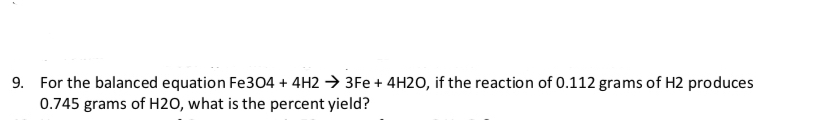# For the balanced equation Fe304 + 4H2 3Fe+ 4H20, if the reaction of 0.112 grams of H2 produces0.745 grams of H20, what is the percent yield?9.

Question
5 viewshelp_outlineImage TranscriptioncloseFor the balanced equation Fe304 + 4H2 3Fe+ 4H20, if the reaction of 0.112 grams of H2 produces 0.745 grams of H20, what is the percent yield? 9. fullscreen
check_circle

Step 1

The balanced chemical equation is shown as,

Step 2

The percent yield is calculated based on the actual yield and theoretical yield. The actual yield of water is given as 0.745 grams. Hence to calculate theoretical yield of water, it is required to calculate the number of moles of water produced when 0.112 grams of hydrogen will react.

Step 3

Hence the moles of water produced is 0.056 and ...

### Want to see the full answer?

See Solution

#### Want to see this answer and more?

Solutions are written by subject experts who are available 24/7. Questions are typically answered within 1 hour.*

See Solution
*Response times may vary by subject and question.
Tagged in

### Chemistry